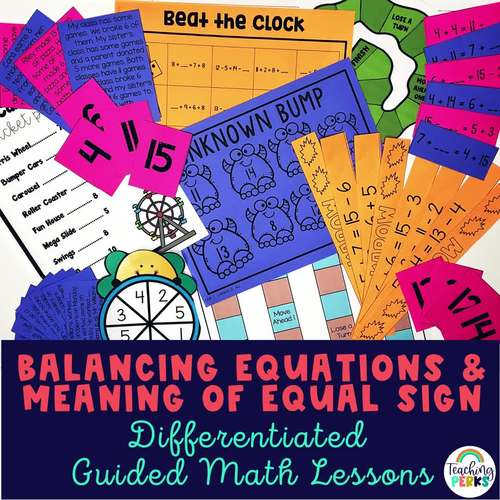# 1st Grade Balancing Equations & Fill in the Missing Number Activities & Lessons;
1st
Subjects
Resource Type
Standards
Formats Included
• PDF
Pages
365 pages
\$14.50
\$14.50
Report this resource to TPT
##### Also included in
1. Set your year up for success with this full-year 1st grade math curriculum! These ready to use math resources come with 14 units that provide differentiated small group math instruction for 1st graders.  Having engaging and fun 1st grade math centers will allow your students to learn and thrive in y
Price \$92.40Original Price \$130.75Save \$38.35

### Description

Do you need fun and engaging resources for balancing equations to improve your math lessons? These 1st grade small group guided math lesson plans will help you teach students how to balance equations and understand the meaning of the equal sign. These LOW PREP fill in the missing number activities will leave your students begging you for more math time!

These math 1st grade balancing equations activities, games, and lessons are differentiated to teach your students exactly what they need to know in order to master standards 1.OA.D.7, 1.OA.D.8, and 1.OA.B.3 in no time! Use these fill in the missing number activities to differentiate for on, above and below level math small group plans.

Included with these 1st grade balancing equations lesson plans:

• 16 lesson plans
• Differentiated two-sided work mats
• Teacher work mats to model with
• Differentiated independent practice pages
• Games with each lesson
• Questions to ask during lessons
• Challenges at the end of each lesson
• Differentiated word problems to use in each lesson
• Answer keys for independent practice pages

Use the materials in these guided math lessons during your small group time and then spiral review them in morning work, centers, homework, assessment, etc. throughout the year to continue to develop mastery in balancing equations and fill in the missing number questions!

❤️Your students will LOVE using these fill in the missing number games in small groups or math centers. Plus, they will gain a deeper understanding of how to balance equations from the independent practice pages that they can complete after the small group table to show what they know.

❤️Reasons to LOVE these 1st grade guided math lessons!❤️

1. They are differentiated! There are differentiated work mats, equation cards, independent practice pages and much more for 3 levels of groups.
2. They are meant to be ink friendly and very low prep! Almost every page in these lessons can be printed on color paper or even just plain white paper to save on ink.
3. They are teacher friendly! Prep consists of printing the lesson plan, work mats front to back for each group, and games. Use dry erase pocket sleeves with the work mats and laminate the games and then save these materials to use year after year!

1st Grade Balancing Equations Lessons Included:

⭐️Different Parts, Same Total

⭐️True or False Subtraction Equations

⭐️True or False Addition and Subtraction Equations Mixed

⭐️True or False Addition on both sides of the equal sign

⭐️True or False Subtraction on both sides of the equal sign

⭐️True or False Addition and Subtraction Mixed Review

⭐️True or False Addition and Subtraction on each side of the equal sign

⭐️Identifying Number Partners

⭐️Missing Number in Subtraction Problems

⭐️Missing Number in Addition and Subtraction Mixed Review

⭐️Missing Number to Balance Addition Equations

⭐️Missing Number to Balance Equations Subtraction

⭐️Missing Number to Balance Addition and Subtraction Equations Mixed Review

⭐️Fill in the Missing Number to Balance One Addition and One Subtraction Equation

OR

✅ Try a free lesson from unit 1 here!

You may also like:

↬Don't forget to leave feedback. You will receive TPT credits that can be used on future purchases!

Want FREE Resources?

Connect with me:

Total Pages
365 pages
N/A
Teaching Duration
N/A
Report this resource to TPT
Reported resources will be reviewed by our team. Report this resource to let us know if this resource violates TPT’s content guidelines.

### Standards

to see state-specific standards (only available in the US).
Use addition and subtraction within 20 to solve word problems involving situations of adding to, taking from, putting together, taking apart, and comparing, with unknowns in all positions, e.g., by using objects, drawings, and equations with a symbol for the unknown number to represent the problem.
Apply properties of operations as strategies to add and subtract. If 8 + 3 = 11 is known, then 3 + 8 = 11 is also known. (Commutative property of addition.) To add 2 + 6 + 4, the second two numbers can be added to make a ten, so 2 + 6 + 4 = 2 + 10 = 12. (Associative property of addition.)
Understand the meaning of the equal sign, and determine if equations involving addition and subtraction are true or false. For example, which of the following equations are true and which are false? 6 = 6, 7 = 8 - 1, 5 + 2 = 2 + 5, 4 + 1 = 5 + 2.
Determine the unknown whole number in an addition or subtraction equation relating three whole numbers. For example, determine the unknown number that makes the equation true in each of the equations 8 + ? = 11, 5 = ▯ - 3, 6 + 6 = ▯.